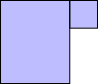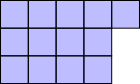#### You may also like### Arithmagons Poster

Arithmagons Poster### Squares in Rectangles Poster

Squares In Rectangles Poster### Isosceles Triangles Poster

Isosceles Triangles Poster - February 2005

# Take Three from Five Poster

##### Age 11 to 14Challenge Level

Yes, any set of five whole numers will always include three numbers which add up to a multiple of 3.

Why?

Imagine trying to find five whole numbers which don't include three numbers that add up to a multiple of 3.

Three multiples of 3 add up to a multiple of 3, so we can use maximum two multiples of 3.

Three numbers that are 1 more than a multiple of 3 add up to a multiple of 3.
To represent a number 1 more than a multiple of 3:e.g. 13Three numbers that are 1 more than a multiple of 3:So we can use maximum two numbers that are 1 more than a multiple of 3.

Three numbers that are 2 more than a multiple of 3 add up to a multiple of 3:So we can use maximum two numbers that are 2 more than a multiple of 3.

So we could have two multiples of 3, two that are 1 more than multiples of 3, and one that is 2 more than a multiple of 3 (or a different arrangement of 2, 2, 1).

However, that will always include at least one of each type of number. Those add up to a multiple of 3: